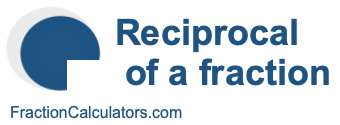Reciprocal of 6/6What is the reciprocal of 6/6? Here we will define reciprocal of 6/6, and show you how to calculate the reciprocal of 6/6 in fraction form and decimal form.

The reciprocal of 6/6 is a fraction or a number that when multiplied by 6/6 is equal to 1. To get the reciprocal of 6/6 based on that definition, we can make the following equation where "R" is the reciprocal of 6/6.

(6/6) × R = 1

When we solve for R, we get the answer to the reciprocal of 6/6 as a fraction as follows:

(6/6) × R = 1
R = 6/6
Reciprocal of 6/6 = 6/6

You can check that the answer is correct by confirming that 6/6 times the reciprocal of 6/6 is equal to 1, like this:

6/6 × 6/6 = 1

To find the decimal answer to the reciprocal of 6/6, you divide the numerator of the reciprocal by the demoninator of the reciprocal, like this:

6 ÷ 6 = 1
Reciprocal of 6/6 = 1

Tip: As you may have inferred from our tutorial above, you can quickly get the reciprocal of any fraction, such as 6/6, by switching the numerator and the denominator.

Reciprocal of a fraction
Do you need the reciprocal of another fraction? No problem! Please enter another fraction below.

Reciprocal of /

Reciprocal of 6/7
Here is the next fraction on our list that we have calculated the reciprocal of.